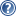###LON-CAPA Help

With variables, you can store strings or numbers. Functions allow you to manipulate these strings or numbers. Functions work like mathematical functions: They take some number of arguments in, and return one argument, usually a number or a string for our purposes. There are a lot of functions available in LON-CAPA. You can see a complete list in the Script sectionand LON-CAPA function section.

For now, let's just look at some simple examples.

\$a = -3.0;

\$b = &sin(\$a);

\$c = &pow(3.0, &abs(\$a));

Figure 1: Some Function Calls

In the Script block, function names start with &. Some example function calls are shown above. You can see that functions can take either variables, numbers, or the results of other function calls as parameters. The &sin function returns the sine of an angle expressed in radians. &pow raises the first parameter to the power of the second parameter. &abs returns the absolute value of the argument.